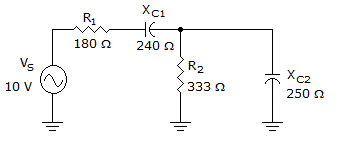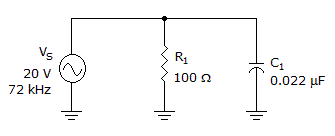# Electronics - RC Circuits

Exercise : RC Circuits - General Questions
11.What is the current through XC1 in the given circuit?

32.2 mA
16 mA
12 mA
48 mA
Explanation:
No answer description is available. Let's discuss.

12.What is the total current in the given circuit?

0.28 A
0.399 A
909 A
0.2 A
Explanation:
No answer description is available. Let's discuss.

13.
Which statement about a series RC circuit is true?
The capacitor's voltage drop is in phase with the resistor's voltage drop.
The current leads the source voltage.
The current lags the source voltage.
The resistor voltage lags the current.
Explanation:
No answer description is available. Let's discuss.

14.If the frequency increases in the given circuit, how would the total current change?

The total current would increase.
The total current would decrease.
The total current would remain the same.
More information is needed in order to predict how the total current would change.